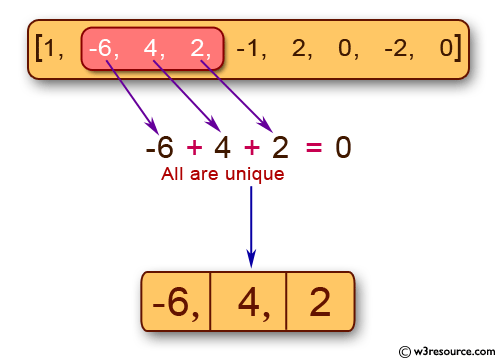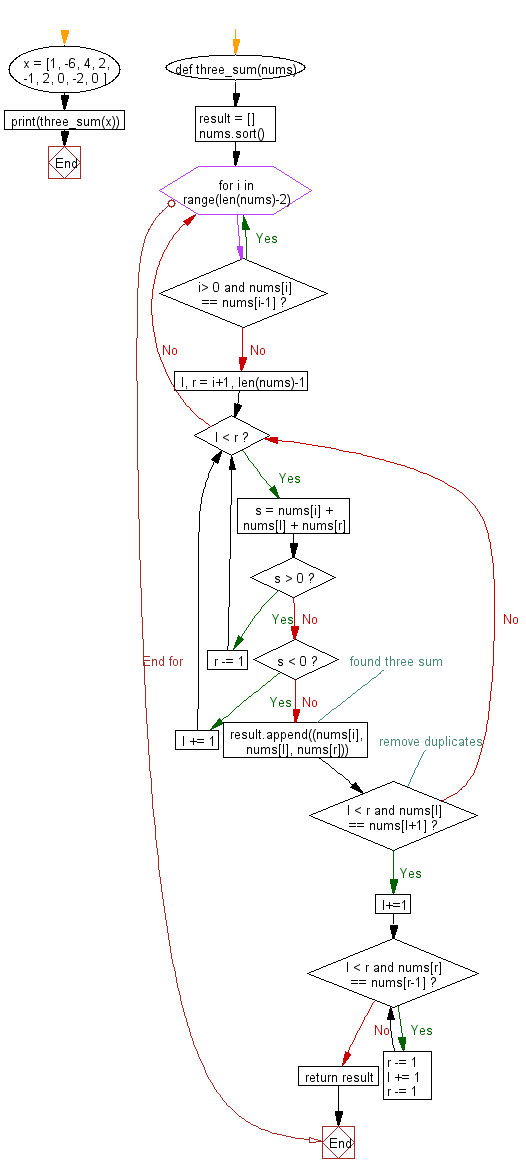﻿ Python: Find unique triplets whose three elements gives the sum of zero from an array of n integers - w3resource# Python: Find unique triplets whose three elements gives the sum of zero from an array of n integers

## Python Basic - 1: Exercise-4 with Solution

Write a Python program to find unique triplets whose three elements gives the sum of zero from an array of n integers.

Pictorial Presentation:Sample Solution:

Python Code :

``````def three_sum(nums):
result = []
nums.sort()
for i in range(len(nums)-2):
if i> 0 and nums[i] == nums[i-1]:
continue
l, r = i+1, len(nums)-1
while l < r:
s = nums[i] + nums[l] + nums[r]
if s > 0:
r -= 1
elif s < 0:
l += 1
else:
# found three sum
result.append((nums[i], nums[l], nums[r]))
# remove duplicates
while l < r and nums[l] == nums[l+1]:
l+=1
while l < r and nums[r] == nums[r-1]:
r -= 1
l += 1
r -= 1
return result

x = [1, -6, 4, 2, -1, 2, 0, -2, 0 ]
print(three_sum(x))
``````

Sample Output:

```[(-6, 2, 4)]
```

Flowchart:## Visualize Python code execution:

The following tool visualize what the computer is doing step-by-step as it executes the said program:

Python Code Editor :

Have another way to solve this solution? Contribute your code (and comments) through Disqus.

What is the difficulty level of this exercise?

Test your Programming skills with w3resource's quiz.

﻿

## Python: Tips of the Day

How to make a flat list out of list of lists?

Given a list of lists l

```flat_list = [item for sublist in l for item in sublist]
```

which means:

```flat_list = []
for sublist in l:
for item in sublist:
flat_list.append(item)
```

is faster than the shortcuts posted so far. (l is the list to flatten.) Here is the corresponding function:

flatten = lambda l: [item for sublist in l for item in sublist]

As evidence, you can use the timeit module in the standard library:

```\$ python -mtimeit -s'l=[[1,2,3],[4,5,6], , [8,9]]*99' '[item for sublist in l for item in sublist]'
10000 loops, best of 3: 143 usec per loop
\$ python -mtimeit -s'l=[[1,2,3],[4,5,6], , [8,9]]*99' 'sum(l, [])'
1000 loops, best of 3: 969 usec per loop
\$ python -mtimeit -s'l=[[1,2,3],[4,5,6], , [8,9]]*99' 'reduce(lambda x,y: x+y,l)'
1000 loops, best of 3: 1.1 msec per loop
```

Explanation: the shortcuts based on + (including the implied use in sum) are, of necessity, O(L**2) when there are L sublists -- as the intermediate result list keeps getting longer, at each step a new intermediate result list object gets allocated, and all the items in the previous intermediate result must be copied over (as well as a few new ones added at the end). So, for simplicity and without actual loss of generality, say you have L sublists of I items each: the first I items are copied back and forth L-1 times, the second I items L-2 times, and so on; total number of copies is I times the sum of x for x from 1 to L excluded, i.e., I * (L**2)/2.

The list comprehension just generates one list, once, and copies each item over (from its original place of residence to the result list) also exactly once.

Ref: https://bit.ly/3dKsNTR